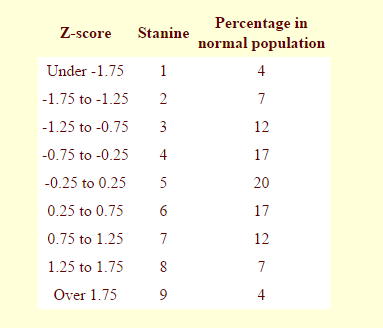# Z-Scores and StaninesZ-Score

A common statistical way of standardising data on one scale so a comparison can take place is using a z-score. The z-score is like a common measure for all types of data. Each z-score corresponds to a point in a normal distribution.

To find a z-score for a specific result in a group of results:

First, calculate the Mean for the group. Then calculate the Standard Deviation. Finally, calculate the z-score using the formula: (student result - Mean) / Standard Deviation.

Stanine

Stanine scores are used in education to compare student performance over a normal distribution.

Stanine scores convert raw test scores to a one-digit whole number to simplify test interpretation.

Typically, stanine scores between 4 and 6 are considered average, scores of 3 or less are below average while scores of 7 or greater are above average.